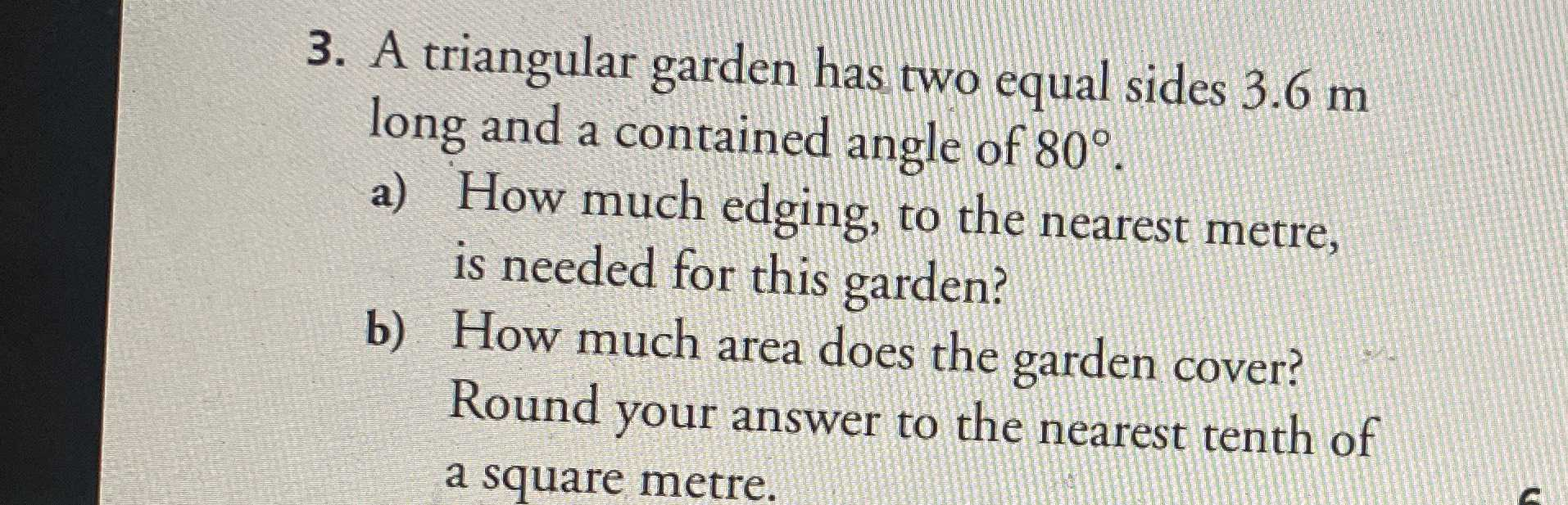### Still have math questions?

Trigonometry
QuestionA triangular garden has two equal sides $$3.6 m$$ long and a contained angle of $$80 ^ { \circ }$$

a) How much edging, to the nearest meter, is needed for this garden?

b) How much area does the garden cover? Round your answer to the nearest tenth of a square meter.